# 深入解析JavaScript编程中的this关键字使用

JavaScript 里的 this 到底指得是什么？很多人都会告诉你 this 指的是当前对象。这样理解对么？在大多数情况下确实没错。比如我们经常会在网页上写这样的 JavaScript：

```<input type="submit" value="提交" onclick="this.value='正在提交数据'" />
```

```var foo = function() {
console.log(this);
}
foo();
new foo();
```

```<input type="button" id="aButton" value="demo" onclick="" />
<script type="text/javascript">
function demo() {
this.value = Math.random();
}
</script>
```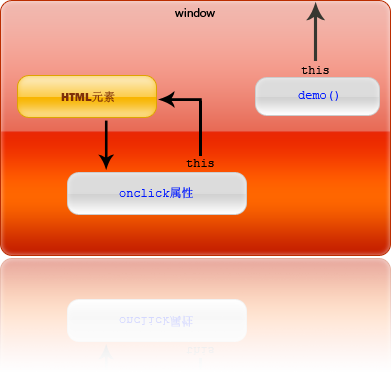```document.getElementById("aButton").onclick = demo;
```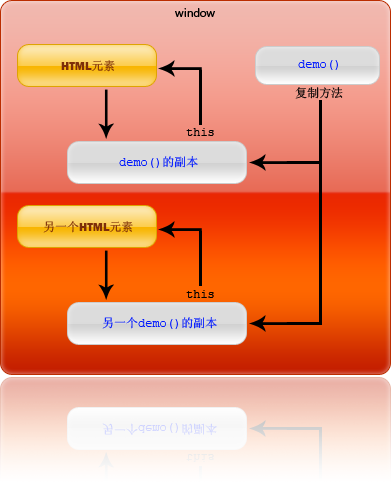```<input type="button" id="aButton" value="demo" onclick="demo()" />
```

```<input type="button" id="aButton" value="demo" />
<script type="text/javascript">
var button = document.getElementById("aButton");
function demo() {
this.value = Math.random();
}
button.onclick= demo;
</script>
```

```function demo() {
this.value = Math.random();
}
```

```<input type="button" id="aButton" value="demo" onclick="demo()" />
<script type="text/javascript">
var button = document.getElementById("aButton");
function demo() {
this.value = Math.random();
}
</script>
```

```function onclick() {
demo();
}
``````<script type="text/javascript">
function demo(obj) {
obj.value = Math.random();
}
</script>
<input type="button" value="demo" onclick="demo(this)" />
<input type="button" value="demo" onclick="demo(this)" />
<input type="button" value="demo" onclick="demo(this)" />
```

this的指向
JavaScript由于其在运行期进行绑定的特性，JavaScript 中的 this 可以是全局对象、当前对象或者任意对象，这完全取决于函数的调用方式。JavaScript 中函数的调用有以下几种方式：作为对象方法调用，作为函数调用，作为构造函数调用，和使用 apply 或 call 调用。常言道，字不如表，表不如图。为了让人更好的理解JavaScript this 到底指向什么？下面用一张图来进行解释：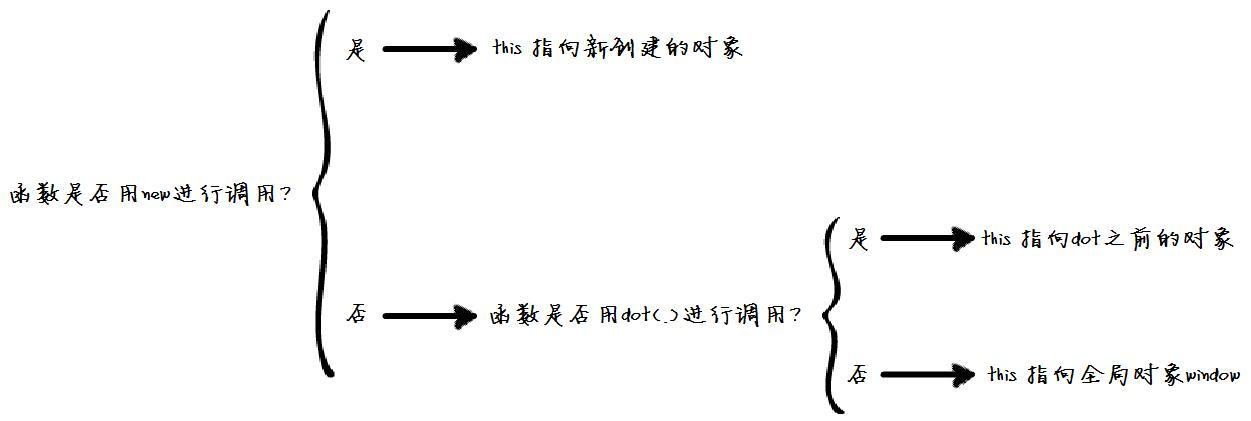```var point = {
x : 0,
y : 0,
moveTo : function(x, y) {
this.x = this.x + x;
this.y = this.y + y;
}
};
//决策树解释：point.moveTo(1,1)函数不是new进行调用，进入否决策，
//是用dot(.)进行调用，则指向.moveTo之前的调用对象，即point
point.moveTo(1,1); //this 绑定到当前对象,即point对象
```

point.moveTo（）函数在 “JavaScript this决策树“中进行判定的过程是这样的：

1）point.moveTo函数调用是用new进行调用的么？这个明显不是，进入“否”分支，即函数是否用dot(.)进行调用？；

2）point.moveTo函数是用dot(.)进行调用的，即进入“是”分支，即这里的this指向point.moveTo中.之前的对象point;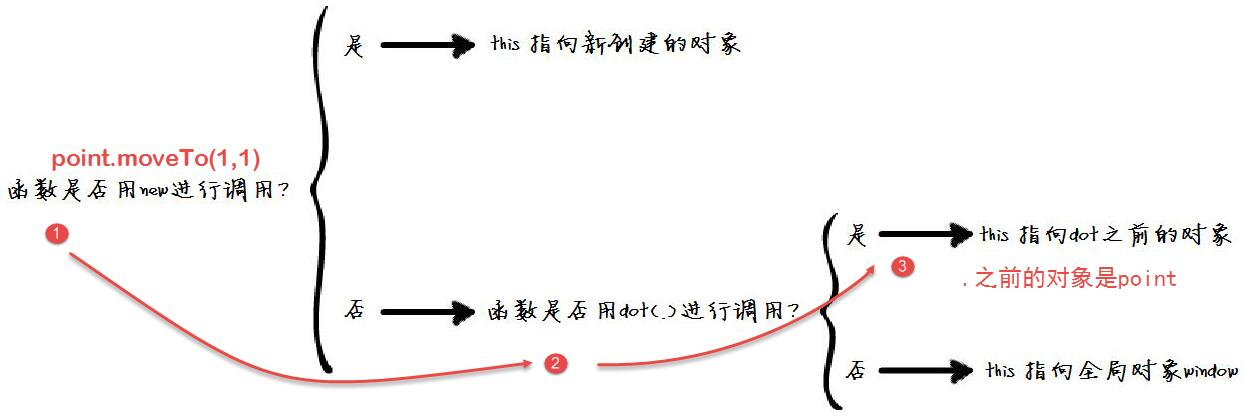```function func(x) {
this.x = x;
}
func(5); //this是全局对象window，x为全局变量
//决策树解析：func()函数是用new进行调用的么？为否，进入func()函数是用dot进行调用的么？为否，则 this指向全局对象window
x;//x => 5
```

func（）函数在 “JavaScript this决策树“中进行判定的过程是这样的：

1）func(5)函数调用是用new进行调用的么？这个明显不是，进入“否”分支，即函数是否用dot(.)进行调用？；

2）func(5)函数不是用dot(.)进行调用的，即进入“否”分支，即这里的this指向全局变量window，那么this.x实际上就是window.x;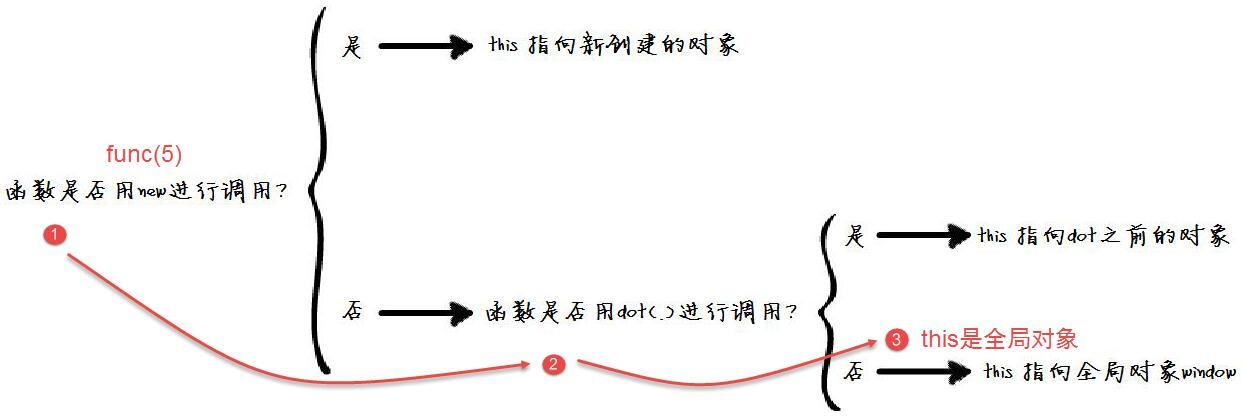```var point = {
x : 0,
y : 0,
moveTo : function(x, y) {
// 内部函数
var moveX = function(x) {
this.x = x;//this 指向什么？window
};
// 内部函数
var moveY = function(y) {
this.y = y;//this 指向什么？window
};
moveX(x);
moveY(y);
}
};
point.moveTo(1,1);
point.x; //=>0
point.y; //=>0
x; //=>1
y; //=>1
```

point.moveTo(1,1)函数实际内部调用的是moveX()和moveY()函数, moveX()函数内部的this在 “JavaScript this决策树“中进行判定的过程是这样的：

1）moveX(1)函数调用是用new进行调用的么？这个明显不是，进入“否”分支，即函数是否用dot(.)进行调用？；

2）moveX(1)函数不是用dot(.)进行调用的，即进入“否”分支，即这里的this指向全局变量window，那么this.x实际上就是window.x;

```function Point(x,y){
this.x = x; // this ?
this.y = y; // this ?
}
var np=new Point(1,1);
np.x;//1
var p=Point(2,2);
p.x;//error, p是一个空对象undefined
window.x;//2
```

Point(1,1)函数在var np=new Point(1,1)中的this在 “JavaScript this决策树“中进行判定的过程是这样的：

1）var np=new Point(1,1)调用是用new进行调用的么？这个明显是，进入“是”分支，即this指向np；

2）那么this.x=1，即np.x=1；

Point(2,2)函数在var p= Point(2,2)中的this在 “JavaScript this决策树“中进行判定的过程是这样的：

1）var p= Point(2,2)调用是用new进行调用的么？这个明显不是，进入“否”分支，即函数是否用dot(.)进行调用？；

2）Point(2,2)函数不是用dot(.)进行调用的？判定为否，即进入“否”分支，即这里的this指向全局变量window，那么this.x实际上就是window.x;

3）this.x=2即window.x=2.

```function Point(x, y){
this.x = x;
this.y = y;
this.moveTo = function(x, y){
this.x = x;
this.y = y;
}
}

var p1 = new Point(0, 0);
var p2 = {x: 0, y: 0};
p1.moveTo.apply(p2, [10, 10]);//apply实际上为p2.moveTo(10,10)
p2.x//10

```

p1.moveTo.apply(p2,[10,10])函数在 “JavaScript this决策树“中进行判定的过程是这样的：

1）p2.moveTo(10,10)函数调用是用new进行调用的么？这个明显不是，进入“否”分支，即函数是否用dot(.)进行调用？；

2）p2.moveTo(10,10)函数是用dot(.)进行调用的，即进入“是”分支，即这里的this指向p2.moveTo(10,10)中.之前的对象p2,所以p2.x=10;

“JavaScript 中的函数既可以被当作普通函数执行，也可以作为对象的方法执行，这是导致 this 含义如此丰富的主要原因。一个函数被执行时，会创建一个执行环境（ExecutionContext），函数的所有的行为均发生在此执行环境中，构建该执行环境时，JavaScript 首先会创建 arguments变量，其中包含调用函数时传入的参数。接下来创建作用域链。然后初始化变量，首先初始化函数的形参表，值为 arguments变量中对应的值，如果 arguments变量中没有对应值，则该形参初始化为 undefined。如果该函数中含有内部函数，则初始化这些内部函数。如果没有，继续初始化该函数内定义的局部变量，需要注意的是此时这些变量初始化为 undefined，其赋值操作在执行环境（ExecutionContext）创建成功后，函数执行时才会执行，这点对于我们理解 JavaScript 中的变量作用域非常重要，鉴于篇幅，我们先不在这里讨论这个话题。最后为 this变量赋值，如前所述，会根据函数调用方式的不同，赋给 this全局对象，当前对象等。至此函数的执行环境（ExecutionContext）创建成功，函数开始逐行执行，所需变量均从之前构建好的执行环境（ExecutionContext）中读取。”

JavaScript编程的单例设计模讲解

JavaScript中利用各种循环进行遍历的方式总结